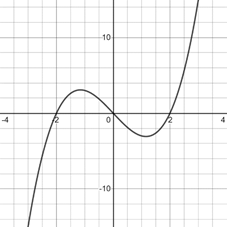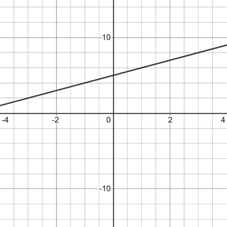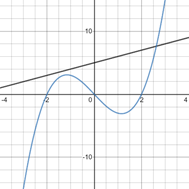# To graph : The equations y = x 3 − 4 x , y = x + 5 in the viewing rectangle [ − 4 , 4 ] by [ − 15 , 15 ] and identify the intersection points.### Precalculus: Mathematics for Calcu...

6th Edition
Stewart + 5 others
Publisher: Cengage Learning
ISBN: 9780840068071### Precalculus: Mathematics for Calcu...

6th Edition
Stewart + 5 others
Publisher: Cengage Learning
ISBN: 9780840068071

#### Solutions

Chapter 1.9, Problem 26E
To determine

## To graph: The equations y=x3−4x,y=x+5 in the viewing rectangle [−4,4]by[−15,15] and identify the intersection points.

Expert Solution

### Explanation of Solution

Given information:

The equations y=x34x,y=x+5 and viewing rectangle [4,4]by[15,15] .

Graph:

The graph of the equation y=x34x can be sketched using the table,

 x y 0 0 2 0 −1 3

The graph of equation is provided below,The graph of the equation y=x+5 can be sketched using the table,

 x y 0 5 2 7 −2 3 −1 4

The graph of equation is provided below,The graph of both equations in same figure is provided below,From the graph it looks as the graphs intersect at 1 point.

Thus, the graphs intersect in the given viewing rectangle. There are 1 intersection points.

Interpretation:

The graph of an equation in a viewing screen is a viewing rectangle.

The x -values to range from a minimum value of xmin =a to a maximum value of xmax =b

The y -values to range from minimum value of ymin =c to a maximum value of ymax =d

Then, the display portion of the graph lies in the rectangle [a,b]×[c,d]={(x,y)|axb,cyd}

The equations y=x34x,y=x+5 are intersect at 1 point in viewing rectangle [4,4]by[15,15] .

### Have a homework question?

Subscribe to bartleby learn! Ask subject matter experts 30 homework questions each month. Plus, you’ll have access to millions of step-by-step textbook answers!